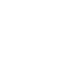# SAT Question of the Day (ACT too!): Jan. 28, 2014

If you are reading this in an email you received from me, do not click the link to sat.collegeboard.org below. Use the link to my website that is farther down on the email.

http://sat.collegeboard.org/practice/sat-question-of-the-day?questionId=20140128&oq=1 (This link takes you to today’s question. If you use my archive, you will see the question related to my SAT explanation for that date.)

The answer is D.  Why are only 61% of the students who have tried this question getting it correct?  Maybe it is because they’ve turned a relatively easy question into a hard one.

I suspect most students have tried to do it as an algebra question (as it is explained by the SAT staff) and made a calculation error.  That is certainly what happens on test day–students are in a hurry and they make a silly mistake.  Let me show you an easy method for avoiding such errors.  Let’s change the algebra into 4th grade arithmetic!

I call this strategy a few different things but they all amount to the same thing: 1) change the algebra into arithmetic, 2) change the abstract into the concrete, or 3) change the variables into numbers.  When the test writer gives you an unknown variable (in this case, x), just follow the rule for x and “substitute a real number.”  Instead of “x bolts per hour,” let’s use 2 instead of x; so, we have “2 bolts per hours” for Machine X and “2 + 6” or “8 bolts per hour” for Machine Y.  We can add 2 + 8 which gives us 10 bolts per hour for the two machines together.  We then multiply 4 times 10 and get 40 bolts in four hours.

Let’s take a look at the answers.  There aren’t any x‘s in the answers because we substituted 2 for x!  Which answer gives us 40 when we have a 2 instead of the x‘s?  For example, Answer A becomes 4(2) + 12 = 20; that’s not 40.  Eliminate A.  When you get to Answer D: 8(2) + 24 = 40, you found the right answer; bubble it in and move on.

I’m awfully good at algebra and could have done this question either way but I always avoid algebra whenever I can on test day because I don’t want to make any silly mistakes.  Doing arithmetic instead assures that I will get this kind of question correct.

You need to practice both ways: the algebra teacher way and the Wizard’s strategy of changing the algebra to arithmetic.  Practicing will help you decide which one works better for you and will raise your confidence.  Confidence is going to raise your score on test day.  It’s time to get to work!

Let’s see what the ACT folks have for us today.

The answer is F.  They tell you in the introductory information, “the student placed a stick vertically into the ground so that 1 meter of its length...”  That is, the length of the stick was constant.  That’s Answer F and all the other answers disagree with the given information.  Be sure you are using the same strategies for the science reasoning test that you have learned for the reading test!  Yes, it’s that simple.

If you use PICK to choose the best answer, your score will improve.  For example, look at this question and the answers.  Answer F is insertable because it fits into the passage without adding or disagreeing with the information you are given.  Answers G, H and J all disagree with the given information which makes them wrong.  That sure is easy.

Do you know what the most important thing is to learn from this question?  First, you’ll never predict the science knowledge that will be on the test; so don’t worry about reviewing science information as part of your test prep.  Second, they’ll explain the science on the test which is all the more reason to not review science topics.  Third, this is a science reasoning test.  You need to apply scientific principles and the most important skill is analyzing and interpreting data.  That is what you need to practice prior to the test.  Get your hands on some actual ACT Science Tests and study the way the test writers present data and ask questions about the charts and graphs in those tests–the more the better.  Work on your speed because that is the major challenge of the test.  Be sure to review the Science test strategies on my free website or Video #10.  Let’s get to work!  Only practice will raise your score.

Remember at the end of the day that you only get to live this day once.  When you go to bed tonight, what will be your answer to the question, “Did I take advantage of my time today?”  I hope you like your answer.

The SAT & ACT Wizard